# How to Manage Your Excel Formulas: Copy, Paste and Autofill

## How to Manage Your Excel Formulas: Copy, Paste and Autofill

The whole purpose of Excel is to make your life easier. This tutorial will help you build foundational skills for working with them. Formulas are at the heart of Excel.

• Learn how to write your first formula in Microsoft Excel to automate math.
• How to copy and paste a working formula into another cell.
• How to use autofill to rapidly apply formulas to an entire column.

In the spreadsheet below, you can see why Excel is so powerful. The top part of the screenshot shows the formulas behind the scenes, while the bottom screenshot shows what the formulas render.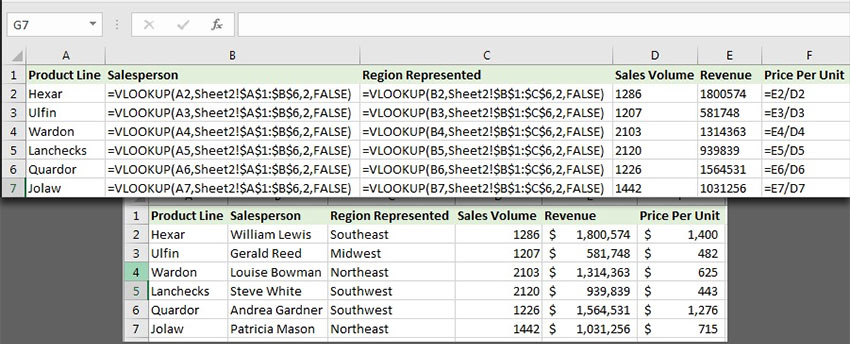This tutorial will teach you how to tame spreadsheets and manage your formulas. Best of all, this tutorial will apply to many spreadsheet apps; whether you use Apple Numbers, Google Sheets, or Microsoft Excel, you’ll find professional tips for working with formulas quickly in a spreadsheet. Let’s get started.

## How to Manage Excel Formulas (Quick Video Tutorial)

Check out the screencast below to learn how to manage your Excel formulas better. I’ll cover everything from how to write your first formula to how to copy and paste them.

## How to Navigate an Excel Spreadsheet

Let’s write our first formula in Microsoft Excel. We write formulas inside of cells, which are the individual boxes in a spreadsheet. Excel is a giant, continuous grid of rows and columns. The place that those rows and columns meet is called a cell.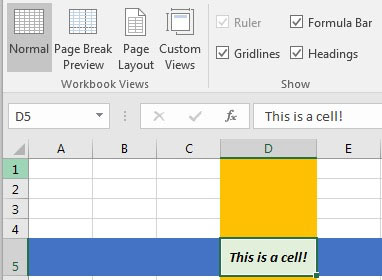Cells are where we can type data, or formulas that work with our data. Each cell has a name that we can refer to when talking about our spreadsheet.

Rows are the horizontal boxes on the left side that are numbered. Columns run from left to right and are lettered. When rows and columns intersect, Excel forms cells. Where column C and row 3 meet, we form a cell referred to as C3.

In the screenshot below, I’ve manually typed the cell names in cells to help you visualize how an Excel spreadsheet works.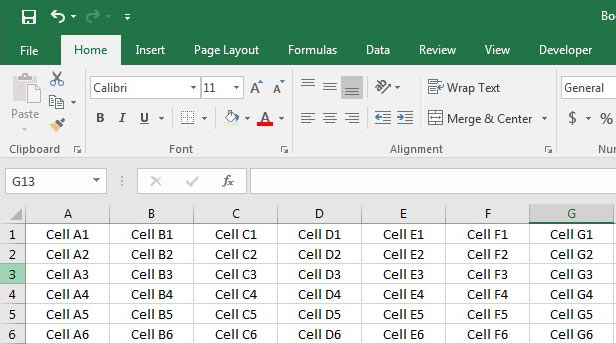Now that we understand the structure of a spreadsheet, let’s move on to writing some Excel formulas and functions.

## Working With Excel Formulas and Functions

Formulas and functions are what we use to work with our typed data. Excel has built-in functions like =AVERAGE to average numbers, while formulas are simple operations like adding two cells together. In practice, the terms are used interchangeably.

For the purpose of this tutorial, I’ll use the term Formula to refer to either of these, since we often work with a combination of both in a spreadsheet.

To write your first formula, double click in a cell and type the = sign. Let’s make our first example extremely simply by adding two values together.

`=4+4`

Check out how this appears in Excel below: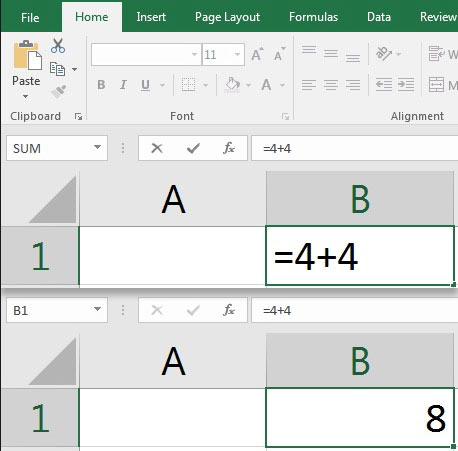After you press Enter on your keyboard, Excel evaluates what you typed. That means that it calculates the formula you typed in, and gives the result. When we’re adding two values, Excel calculates what they sum up to and prints the total.

Notice the Formula Bar that sits above the spreadsheet and shows the formula for adding the two numbers together. The formula is still behind the scenes, even when we’re viewing the output.

The cell contains “=4+4” as a formula, while the spreadsheet prints the result. Try out using similar formulas with subtraction, multiplication (with the * symbol) and division (with the / symbol.) You can type formulas in either the formula bar or in a cell.

This is an extremely simple example, but it illustrates an important concept: behind the cells in your spreadsheet, you can build powerful formulas that work with your data.

### Excel Formulas With Cell References

There are really two ways to work with formulas:

• Use formulas with the data right inside of the formula such as the example we showed above (=4+4)
• Use formulas with cell references to work with data more easily, such as =A2+A3

Let’s look at an example below. In the screenshot below, I’ve logged the list of sales for my company for the first 3 months of 2016. Now, I’ll sum them up by adding the three months of sales together.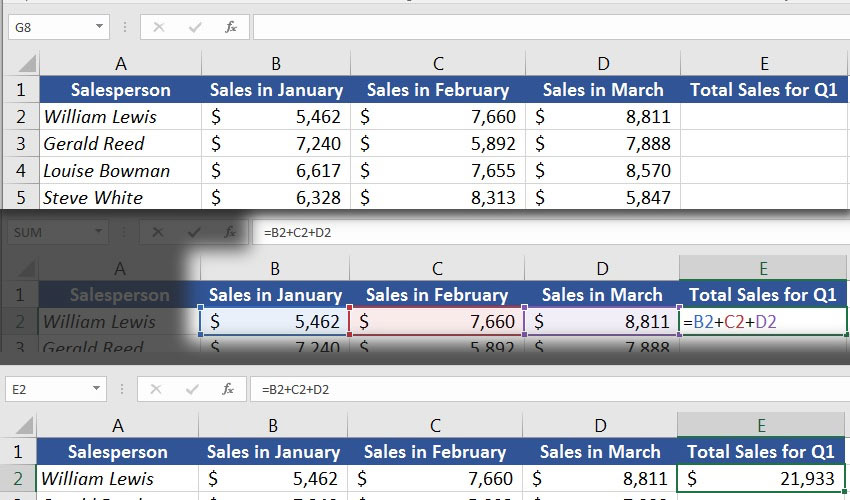In this case, I’m using an inline formula with cell references. I added cells B2, C2, and D2 to get the total of the three months.

The formulas that we worked with are just a few of the available ones in Excel. To keep learning, try out some of these built-in functions in Excel:

• =AVERAGE to average values.
• =COUNT to count up the number of items in a set of data.
• =TODAY to print today’s date in a cell.
• =TRIM to remove spaces from the beginning and end of a cell.

## How to Copy and Paste Formulas in Excel

Now that we’ve written a few formulas in Excel, let’s learn how to copy and paste them.

When we copy and paste a cell with a formula in it, we’re not copying the value – we’re copying the formula. If we paste it in somewhere else, we’re copying the Excel formula over.

In the example below, here are the steps I took:

1. I copied (Ctrl + C) cell E2, which was a formula that added B2, C2, and D2.
2. Then, I selected all of the other cells in column E by clicking and dragging over the column.
3. I pressed (Ctrl + V) to paste the same Excel formula to all of the cells in column E.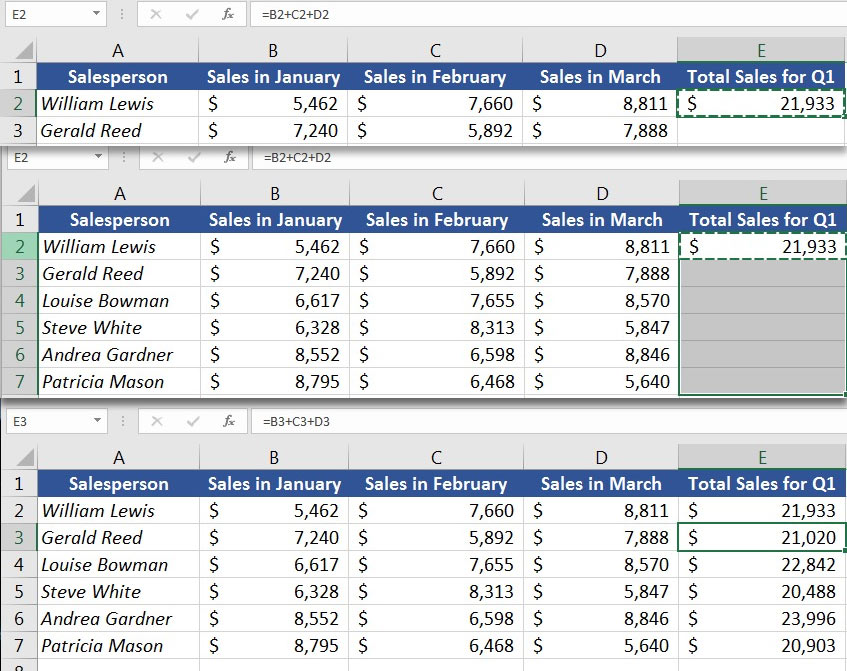As you can see in the screenshot above, pasting the formula doesn’t paste the value (\$21,933). Instead, it pastes a formula.

The formula we copied was in cell E2. It added cells B2, C2, and D2. When we pasted it into E3, for example, it didn’t do the same thing – instead, it added the values from B3, C3, and D3.

Basically, Excel is guessing that you want to add the three cells to the left of the current cell, which is perfect in this case. When you copy and paste a formula, it points to cells with relative references (more on that below) instead of copying and pasting the exact same references.

## How to Autofill Formulas in Excel

Autofilling formulas is one of the fastest ways to expand your formulas. If all of the cells you want to copy your formula to are adjoined, you can autofill formulas in your spreadsheet.

To autofill in Excel, hover over the cell with your formula. As you place your mouse cursor in the lower right corner, you’ll see the cursor turn into a shape that looks like a plus sign. Double click to autofill formulas.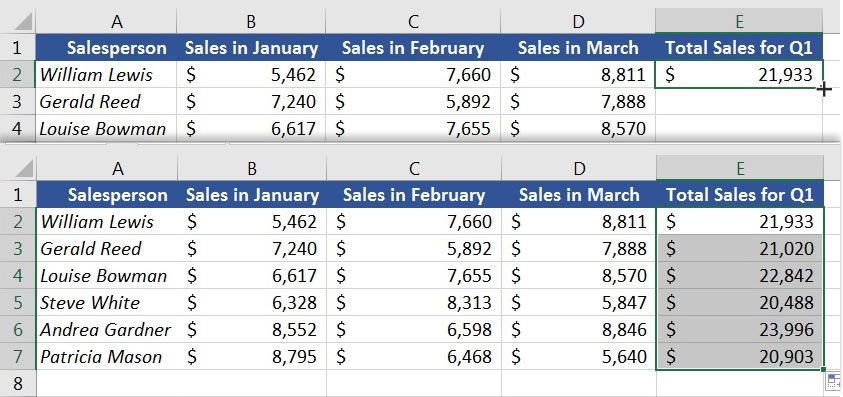Note: An issue to watch-out for when using autofill is that Excel doesn’t always guess perfectly what formulas to fill. Be sure to double-check that autofill puts the correct formulas into your spreadsheet.

## Relative Versus Absolute References

Let’s get a bit more advanced now, and talk about relative versus absolute references in Excel.

In the example where we calculated the quarterly sales totals, we wrote a formula with relative references. That’s why our formula worked correctly when we dragged the formula down. It added the three cells to the left of the cell – not the exact same cells over and over.

Absolute references freeze the cells that we’re referring to. Let’s look at an example of why absolute references are also useful.

Let’s calculate our employees’ sales bonus for the quarter. Based on the sales generated, we’ll calculate a sales bonus of 2.5%. Let’s start off by multiplying the sales times the bonus percentage for our first employee.

I’ve got a box with the bonus percentage, and I’ll multiply the sales times the bonus percentage: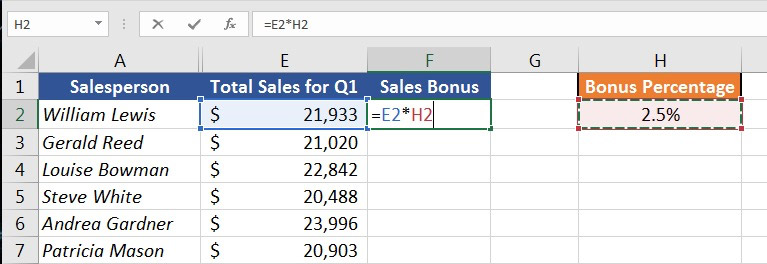Now that we’ve calculated the first sales bonus, let’s drag down the formula to calculate all of the sales bonuses: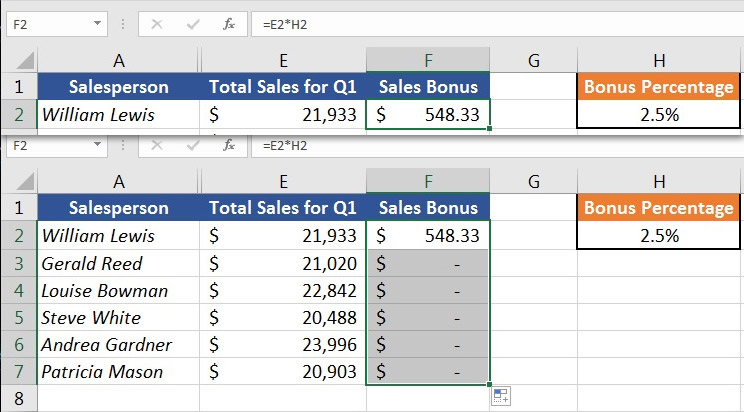However, this didn’t work. Because the bonus percentage is always in the same cell – H2; so the formula doesn’t work when we drag it down. We get zeroes for every cell.

Here’s how Excel attempted to calculate F3 and F4, for example: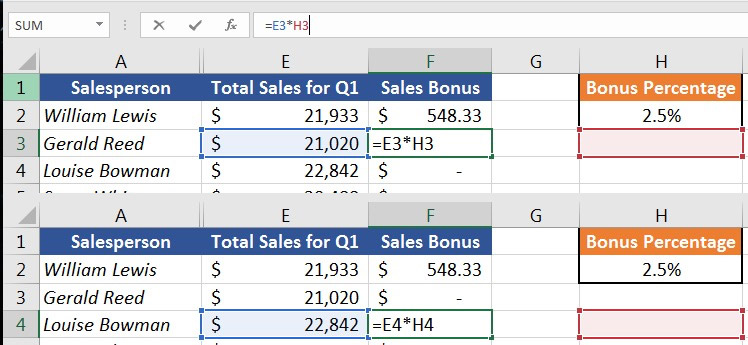Basically, we need to change the formula so that Excel keeps using cell H2 to multiply the bonus amount. This is where we use an absolute reference.

Absolute references tell Excel to freeze the cell that’s used in the formula, and don’t change it as we move the formula around.

Up until now, our formula has been:

`=E2*H2`

To freeze this formula to use cell H2, let’s transform it into an absolute reference:

`=E2*\$H\$2`

Notice that we add dollar signs in the cell reference. This tells Excel that no matter where we put our formula, look back to cell H2 to get the bonus percentage. We leave the “E2” portion unchanged, because as we drag the formula down, we do want the formula to adapt to each employees’ sales.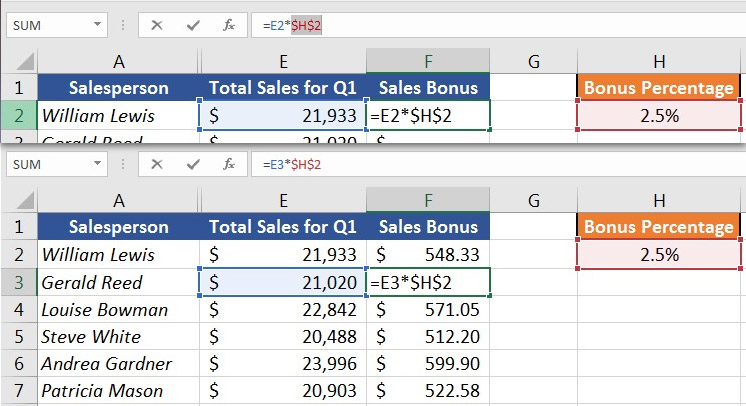Absolute references allow you to really get specific with how your formulas should work. In this case, we used an absolute reference to keep the formula locked on the same bonus percentage for each employee.

## Recap and Keep Learning

Formulas are what make Excel so powerful. Write one formula and drag it down, and you’ve removed manual work for your spreadsheet.

This tutorial was designed as an introduction to working with spreadsheets. We’ve been building out more content recently to help entrepreneurs and freelancers master spreadsheets: